For this basic module we simply take the suitable backbone module of the Hyperscript "Introduction to Materials Science II"This module is the newest and updated version. The module as it existed in Sept. 01 is reprocuced below.

# Ohm's Law and Materials PropertiesIn this subchapter we will give an outline of how to progress from the simple version of Ohm's "Law", which is a kind of "electrical" definition for a black box, to a formulation of the same law from a materials point of view employing (almost) first principles.In other words: The electrical engineering point of view is: If a "black box" exhibits a linear relation between the (dc) current I flowing through it and the voltage U applied to it, it is an ohmic resistor.The (extreme) Materials Science point of view is: Tell me what kind of material is in the black box, and I tell you: If it is an ohmic resistor, i.e. if the current relates linearly to the voltage for reasonable voltages and both polarities; and What its (specific) resistance will be, including its temperature dependence. And everything else of interest.Lets see what we have to do for this approach.

1. Step: Move to specific quantitiesFirst we switch from current I and voltage U to the current density j and the field strength E, which are not only independent of the (uninteresting) size and shape of the body, but, since they are vectors, carry much more information about the system.This is easily seen in the schematic drawing below.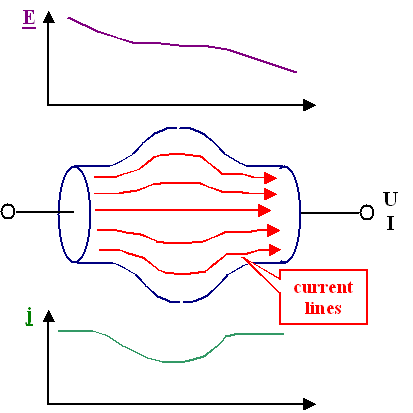Current density j and field strength E may depend on the coordinates, because U and I depend on the coordinates, e.g. in the way schematically shown in the picture to the left. However, for a homogeneous material with constant cross section, we may write

j  =  I
Fwith F = cross sectional area. The direction of the vector j would be parallel to the normal vector f of the reference area considered, so in full splendor we must write

j = I
F
· fThe field strength is
E  =  U
dHere, d = distance between the points at which U is measured. If we want E as a vector, we have, in principle, to solve the Poisson equation:
Ñ· E  =   r
ee0With r = charge density. For the homogeneous case E is parallel to f again which is clear without calculation.We now write down Ohm's law with the new quantities and obtain
j · F  =  I  = 1
R
· U  =  1
R
· (E · d)
j  =  d
F · R
· EThe fraction d / (F · R) obviously (think about it!) has the same numerical value for any homogeneous cube (or ....) of a given material; it is, of course, the specific conductance or, for short, the conductivity s:
s  =  1
r
=  d
F · Rand r is the specific resistance or, for short, the resistivity.Of course, we will never mix up the resistivity r with the charge density r or general densities r, because we know from the context what is meant!The resistivity obtained in this way is of course identical to what you would define as specific resistance by looking at some rectangular body with cross-sectional area F and length d.Being specifc quantities, conductivity has the dimension [s] = W–1cm–1, and the dimension of resistivity is [r] = Wcm. The latter is more prominent and you should at least have a feeling for representative numbers by remembering this:
r (metal)  »  2 µWcm

r (semicoductor)  »  1 Wcm

r (insulator)  »  1 GWcmRestricting ourselves to isotropic and homogenoeus materials restricts s and r to being scalars with the same numerical value everywhere, and Ohm's law now can be formulated for any material with weird shapes; we "simply" have

j  =  s  · EOhm's law in this vector form, however, is now valid at any point of a body, since we do not have to make assumptions about the shape of the body.Take an arbitrarily shaped body with current flowing through it, cut out a little cube (with your "mathematical" knife) at the coordinates (x,y,z) without changing the flow of current, and you must find that the local current density and the local field strength obey equation given above locally.
j(x,y,z)  =  s  · E(x,y,z)This is a much more powerful version of Ohm's law! Especially, because we now harbor a suspicion: There is no good reason why j must always be parallel to E. This means that for the most general case, conductivity is not a scalar quantity but a tensor; s = sij.
(There is no good way to write tensors in html; we use the ij index to indicate tensor properties.Ohm's law then writes
jx = sxx · Ex + sxy · Ey + sxz · Ez
jy = syx · Ex + syy · Ey + syz · Ez
jz = szx · Ex + szy · Ey + szz · EzFor anisotropic inhomogeneous materials you have to take the tensor, and its components will all depend on the coordinates – that is the most general version of Ohm's law.Note that this is not so general as to be meaningless: We still have the basic property of Ohm's law: The local current density is directly proprotional to the local field strength (and not, for example, to exp(– const. · E).Our goal now is to find a relation that allows to calculate sij for a given material (or material composite); i.e. we are looking forsij = sij(material, temperature, pressure, defects... )

2. Step: Describe sij in Terms of the Carrier PropertiesElectrical current needs mobile charged carriers. We therefore want to express sij in terms of the properties of the carriers present in the material under investigation.To do this, we will look at an electrical current as a "mechanical" stream or current of (charged) particles and compare the result we get with Ohm's law.First, lets define an electrical current in a wire in terms of the carriers flowing through that wire. There are three crucial points to consider1. The external electrical current as measured in an Ampèremeter is the result of the net current flow through any cross section of an (uniform) wire.In other words, the measured current is proportional to the difference of the number of carriers of the same charge sign moving from the left to right through a given cross sectional area minus the number of carriers moving from the right to the left.In short: the net current is the difference of two partial currents flowing in opposite directions: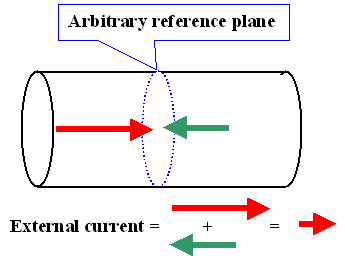We will encounter cases where we have to sum up 8 partial currents to arrive at the externally flowing current, so keep this in mind!2. In summing up the individual current contributions, make sure the signs are correct. The rule is simple:The electrical current is (for historic reasons) defined as flowing from + to . For a particle current this means: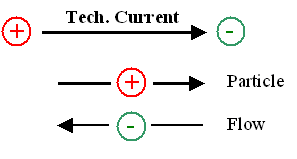In words: A technical current I flowing from + to may be obtained by negatively charged carriers flowing in the opposite direction (from to +), by positively charged carriers flowing in the same direction, or from both kinds of carriers flowing at the same time in the proper directions.The particle currents of differently charged particles then must be added! Conversely, if negatively charged carriers flow in the same directions as positively charged carriers, the value of the partial current flowing in the "wrong" direction must be subtracted to obtain the external current.3. The flow of particles through a reference surface as symbolized by one of arrows above, say the arrow in the +x -direction, must be seen as an average over the x -component of the velocity of the individual particles in the wire. Instead of one arrow, we must consider as many arrows as there are particles and take their average. A more detailed picture of a wire thus looks like this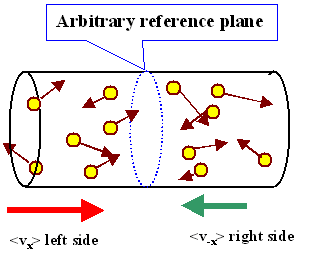If we want to obtain the net flow of particles through the wire (which is obviously proportional to the net current flow), we could take the average of the velocity components <vx> pointing in the +x direction (to the right) on the left hand side, and subtract from this the average <v–x> of the velocity components pointing in the x direction (to the left) on the right hand side.We call this difference the drift velocityof the ensemble of carriers. If there is no driving force, e.g. an electrical field, the velocity vectors are randomly distributed and <vx> = <v-x>; the net current is zero as it should be.Average properties of ensembles can be a bit tricky. Lets look at some properties by considering the analogy of a localized swarm of summer flies "circling" around like crazy, so that the ensemble looks like a small cloud of smoke. A more detailed treatment can be found in the advanced section.First we notice that while the individual fly moves around quite fast, its vector velocity vi averaged over time t, <vi>t, must be zero as long as the swarm as an ensemble doesn't move.In other words, the flies, on average, move just as often to the left as to the right, etc. The net current produced by all flies at any given instance or by one individual fly after sufficient time is obviously zero for any reference surface.In real life, however, the fly swarm "cloud" often moves slowly around – it has a finite drift velocity which must be just the difference between the average movement in drift direction minus the average movement in the opposite direction.The drift velocity thus can be identified as the proper average that gives the net current through a reference plane perpendicular to the direction of the drift velocity.This drift velocity is usually much smaller than the average magnitude of the velocity <v> of the individual flies. Its value is the difference of two large numbers – the average velocity of the individual flies in the drift direction minus the average velocity of theindividual flies in the direction opposite to the drift direction.Since we are only interested in the drift velocity of the ensemble of flies (or in our case, carriers) we may now simplify our picture as follows: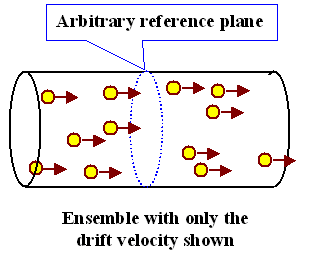We now equate the current density with the particle flux density by the basic law of current flow:Current density j = Number N of particles carrying the charge q flowing through the cross sectional area F (with the normal vector f and |f| = 1) during the time interval t, or
j  =  q · N
F · t
· fIn scalar notation, because the direction of the current flow is clear, we have

j  =  q · N
F · tWith n = N / v = density of carriers in a volume V, and V = F · d with d being the required certain length of the wire needed to obtain V, we now must consider how many carriers contained in the volume V will flow through the reference plane F.The trick is to take
d  =  vD · tThis makes sure that all carriers contained in this length will have reached F after the time t has passed, and thus all carriers contained in the volume V = F· vD · t will contribute to the current density. We can write the current equation as follows:
j  =  q · N
F · t
=  q · n · V
F · t
=  q · n · F · d
F · t
=  q · n · F · vD · t
F · tThis was shown in excessive detail because now we have the fundamtental law of electrical conductivity (in obvious vector form)

j  =  q · n · vDThis is a very general equation relating a particle current (density) via its drift velocity to an electrical current (density) via the charge q carried by the particles.Note that it does not matter at all why an ensemble of charged particles moves on average. You do not need an electrical field as driving force anymore. If a concentration gradient induces a particle flow via diffusion, you have an electrical current, too, if the particles are charged.Of course, if you have different particles, with different density, drift velocity, and charge, you simply sum up the individual current contributions (following the sign convention as pointed out above).All we have to do now is to compare this equation to Ohm's law:
j  = q · n · vD  =  s · EWe then obtain
s  :=  q · n · vD
E
=  constantThis implies by necessity
vD
E
=  constantA simple, but far reaching equation!What it means is that if vD/E = const. holds for any (reasonable) field E, the material will show ohmic behavior. We have a first condition for ohmic behavior expressed in terms of material properties.If, however, vD/E is constant (in time) for a given field, but with a value that depends on E, we have s = s(E); the behavior will not be ohmic!The requirement vD/E = const. for any electrical field requires a constant average velocity in field direction for the particle which is directly proportional to E. This leads to a simple conclusion:Since a constant electrical field exerts a constant force on a charged particle, without some kind of friction its velocity would grow to infinity. We thus conclude that there must exist some mechanism that acts like a frictional force on all accelerated particles, and that this frictional force in the case of ohmic behavior must be in a form where the average drift velocity obtained is proportional to the driving force.Since vD/E = constant must hold for all ohmic materials under investigation, we may give it a name:It is called the mobility m of the carriers, with the unit
[m] = (m/s)/(V/m) = m2/V · s.The mobility m (Deutsch: Beweglichkeit) then is a material constant; it is determined by the "friction", i.e. the processes that determine the average velocity for carriers in different materials subjected to the same force q · E.Thinking ahead a little bit, we realize that m is a material constant even in the absence of electrical fields – it simply expresses how fast carriers give up surplus energy to the lattice; and it does not matter how the got the surplus energy. It is therefore no suprise if m pops up in all kinds of relations, e.g. in the famous Einstein–Smoluchowski equation linking diffusion coefficients and mobility of particles.We now can write down the most general form of Ohm's law applying to all materials meeting the two requirements: n = const. and m = const. everywhere. It is expressed completely in particle (= material) properties:

s  =  q · n · mSinc we like to have s as a positive number, we always take only the magnitude of the charge q carried by a particle.However, if we keep the sign, e.g. write s = –e · n · me for electrons carrying the charge q = –e (e = elementary charge), we now have an indication if the particle current and the electrical current have the same direction (s > 0) or opposite directions s < 0) as in the case of electrons.But it is entirely a matter of taste if you like to schlepp along the signs all the time, or if you like to fill 'em in at the end.Everything more detailed then this is no longer universal but specific for certain materials. The remaining task is to calculate n and m for given materials (or groups of materials).This is not too difficult for simple materials like metals, where we know that there is one (or a few) free electrons per atom in the sample – so we know n to a sufficient approximation. Only m needs to be determined.This is fairly easily done with classical physics; the results, however, are flawed beyond repair: They just do not match the observations and the unavoidable conclusion is that classical physics must not be applied when looking at the behavior of electrons in simple metal crystals or in any other structure – we will show this in the immediately following section 2.1.3.We obviously need to resort to quantum theory and solve the Schrödinger equation for the problem.This, surprisingly, is also fairly easy in a simple approximation. The math is not too complicated; the really difficult part is to figure out what the (mathematical) solutions actually mean. This will occupy us for quite some time.2.2.1 Intrinsic Properties in Equilibrium2.2.2 Doping and Carrier Concentration2.2.4 Simple Junctions and Devices2.3.4. Useful RelationsBasic EquationsFree Electron Gas in Crystals with Unequal DimensionsAlternative Derivation of the Einstein RelationSolution to 3.1.1

© H. Föll (Semiconductor - Script)